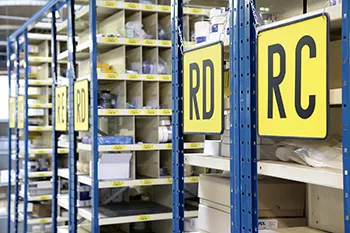There is often discussion about the reading distance of location signs in warehouses. But what is the right font size to ensure readability for each distance? In this blog, we elaborate on this issue using a few practical examples and useful rules of thumb.

##The font size is decisive

The reading distance of a sign is primarily determined by the font size. In addition, the font and the colours used along with the contrast with the background also influence readability.

The following formula is usually used to determine the minimum font size:

H = L/300
Where: H = font size in cm. L = reading distance in cm.

Please note: this formula applies in ideal conditions. Ideal conditions are generally unattainable in a warehouse. This may be because:

• the lighting between the racks is not ideal;
• the location labels on high shelves must be read at an angle;
• the text only takes up a small part of the field of view, which makes for visual distraction.

## Rule of thumb for warehouse use

This is why we advise you use larger fonts in a warehouse, for example on rack labels or location signs. We use the following rule of thumb to determine the ideal font size for a specific distance:

H = L/200
Where: H = font size in cm. L = reading distance in cm.

## Examples

A few examples using the abovementioned rule of thumb for warehouse settings:

• The minimum font size for a location label on the beam of a rack which needs to be read from a distance of about 4 metres would be:
H = 400/200 = 2cm.
• The minimum font size for a location number on a shelf which needs to be read from a distance of about 2 meters would be:
H = 200/200 = 1cm.
• The minimum font size for a location sign on a docking bay which needs to be read from a distance of about 20 meters would be:
H = 2000/200 = 10 cm.